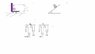# Some questions(pulleys,rotation)

• Dweirdo
In summary, the conversation discusses three different questions related to ratios, acceleration, and energy in a physics context. The first question involves finding the relationship between energy and acceleration, with the person seeking a hint to help solve the problem. The second question is a multiple-choice question about a sphere rotating on an inclined plane, and the person is unsure of the correct answer. The third question involves a system with multiple pulleys, and the person is struggling to find the correct solution.

## Homework Statement

Hi all!
I'm attaching a picture with three diagrams,each for a question(they're numbered in green)
so question number 1:
i had to find all kinds of ratios between the accelerations and stuff, so this was good,
the last part of the question was "Find the acceleration using Energy" , I don't have an idea ow to relate energy to acceleration , It's pretty easy to find using Newton's laws, but not energy, so any hint here could help.
Info:no friction between any surface nor pulleys, neglect mass of pulleys,I defined mass 1 and mass 2 as numbered in sketch.
question number 2:
It's a multiply choice question :
A sphere that rotates around his axis of rotation was put on an inclined plane(long),Center of mass velocity in the moment it was put on the inclined plane was 0 , direction of rotation is as indicated in the sketch.
which of the following is true :
a)there is a period of time that the acceleration is greater than g*sin(beta).
b)there is a period of time that the acceleration is smaller than g*sin(beta).
c)there is a period of time that the acceleration is equal to g*sin(beta).
d)the angular velocity firs decreases then increases.

question number 3:
in the sketch, everything is ideal, the system is released from rest,the distance between M and 2M is:
a)not changing in system number 1, and increasing in system number 2.
b)decreases in system 1, increases in 2.
c)constant in both systems.
d)increases in both.

## Homework Equations

Lots of!
F=ma.
W(conservative)=change in kinetic energy.
W(non conservative) = change in total energy.
rolling no slipping c.O.M velocity V=WR.
Acceleration of a sphere on an inclined plane when rolling no slipping =(2/3)gsin(beta) if i remember correctly.

## The Attempt at a Solution

ok question number 1 left only the last part which is what i wrote, here I have almost no idea, I just found the velocity ratio, and i think I need to attach work of tension and change in PE and Ek some how ... can't find a way, so a hint will help :}
2)
wow this one is annoying , so what I have so far is , the velocity is 0 initially , so it's slipping, till it will have velocity of W*R , so there is acceleration downwards the plane, but i think there should be friction as well, so the answer should be b)period when acceleration is smaller than gsinB? or it's d? :S or else XDD
3)
Truly, that many pulleys make me sick, I would really like to know what steps are involved in this kind of questions, are You doing it in the intuitive way?
well anyway , I'll start from system number 2(left).
0.5M is definitely going up, so either M and 2M going down or only one of them.
in the first system , i think 3M goes down.2M and M going up.
well I'm lost in this question.
any hints/stories/jokes are welcome!
thanks!

#### Attachments

•helpplz.jpg
8.8 KB · Views: 382
Last edited:
bump,any one?

Ok I've got the answer for question 3(constant distance), anyone can help me with the others? :S

Really nobody knows? or It's just cause the thread is to long? XD